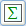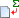The Formula Tool is used to add simple mathematical calculations to your reports. The formula can include database fields and VisualNEO for Windows variables. When using database fields, the results of the calculation may be different depending on where the Formula object is placed on your report. Some types of formulas only make sense when placed on top of certain types of Partitions, while others can be placed anywhere. You can change the object's appearance using the Text Style and Object Style Palettes.

To add formula to your report, use the mouse to draw a rectangle where you would like the object to appear. The Formula Properties screen will be displayed, allowing you to enter your formula.The contents of the Formula object must be a valid mathematical formula. A formula can include mathematical operators (+, -, *, /, MOD), parentheses, functions, numbers, compatible database fields and VisualNEO for Windows variables. Examples of valid mathematical formulas are:

1 + 2

(10 / 2) * 4

[Orders.Qty] * [Orders.UnitPrice]

SUM( [Orders.TotalPrice] )

Database fields must be typed using a special syntax similar to that used to display NeoDBpro field variables in VisualNEO for Windows. As you know, a database can contain one or more tables, which contain records which are composed of fields. When creating or opening a database table, NeoDBpro will automatically create VisualNEO for Windows variables for each field it finds. In Report Designer, each field variable is a combination of the table name, a period (.) and the field name surrounded by square brackets. For example:

[Orders.UnitPrice]

[Orders.Qty]

[Orders.TotalPrice]

Unlike NeoDBpro, Report Designer does not use the database ID as part of the field variable. That's because reports can only work with one database at a time, and since reports exist outside the context of NeoDBpro, the database ID has no meaning.

The Formula object supports the following functions:

 AVG Calculate the average value of a single field from each record in a database table. If a search query is active, only records matching the query will be included in the calculation. For example: AVG( [Orders.UnitPrice] ) COUNT Returns the number of records in a database table. If a query is active, the count will include only those records matching the query. It is not necessary to include the field name portion of the variable for this function. For example: COUNT( [Orders] ) SUM Calculate the sum of a single field from each record in a database table. If a search query is active, only records matching the query will be included in the calculation. For example: SUM( [Orders.TotalPrice] ) MIN Calculate the minimum value of a single field from each record in a database table. If a search query is active, only records matching the query will be included in the calculation. For example: MIN( [Students.Age] ) MAX Calculate the maximum value of a single field from each record in a database table. If a search query is active, only records matching the query will be included in the calculation. For example: MAX( Students.Age] ) INT Returns the integer part of a decimal number. For example: INT( [Orders.SalesTax] ) FRAC Returns the fractional part of a decimal number. For example: FRAC( [Orders.UnitPrice] ) ROUND Converts a decimal value to the nearest whole number. For example: ROUND( [Orders.UnitPrice] ) LOWERCASE Converts a string or database field to lowercase. For example: LOWERCASE( [Clients.Name] ) UPPERCASE Converts a string or database field to uppercase. For example: UPPERCASE( [Clients.Name] ) NAMECASE Capitalize the first letter of each word in a string or database field. For example: NAMECASE( [Clients.Name] )

Note: Functions in red generally will only work when placed onto a Report Summary or Footer type Partition. Placing them elsewhere on a report will yield unpredictable results.

You can use thebutton to select a function. If you have selected a Database Source from the Report Properties screen, you can use thebutton to select from a list of available field names. Enabling the Auto-size option will allow the Formula object to expand or contract if the contents are larger than the object's bounds.

Use the conditional Formatting button to specify alternate font and fill colors to use for this object under certain conditions. The condition statement is similar to that used by VisualNEO for Windows's built-in IfEx action. If the condition evaluates to TRUE then the alternate formatting will be used. If FALSE, then the object's default formatting will be used. For example, the following condition triggers the alternate formatting when the value of the [Orders.UnitPrice] field is greater than or equal to 500:

[Orders.UnitPrice] >= 500

If you want to compare the calculated contents of this object (the results of the formula), you can use the special keyword "Value" in your conditional statement. For example:

Value >= 500

You can use AND and OR operators to construct complex conditional statements. Each element in a conditional statement should be surrounded by parentheses. For example:

(Value >= 500) OR (Value <= 100)

Literal text should be surrounded by single quotation marks. For example:

Value = 'Sunday'

You can also incorporate VisualNEO for Windows variables into the conditional statement. For example:

(Value <= 500) AND ([CheckBox1] = 'Checked')

Formatting Calculations

Calculations can be formatted by adding a pipe character and a special code to the end of the formula. For example, the following will format the results of a calculation with a dollar sign, two digits to the right of the decimal and comma thousands separator:

[Orders.Qty] * [Orders.UnitPrice] |\$#,#.00

When printed, this will produce results similar to the following:

\$1,250.50

See Formatting Fields for more information.